# 问题描述

## Sample Input

``````6 2 3
1 3 5
1 4 7
2 8 12
3 8 4
4 9 12
9 10 2
1 2
8 9 10
``````

## Sample Output

``````9
``````

# 问题分析

Dijkstra算法中设置了一顶点集合S，从源点s到集合中的顶点的最终最短路径的权值均已确定。算法反复选择具有最短路径估计的顶点u∈V-S，并将u加入到S中，对u的所有出边进行松弛。在下面的 算法实现中，用到了顶点的最小优先级列队Q，排序关键字为顶点的d值。

``````DIJKSTRA(G, w, s)
1 INITIALIZE-SINGLE-SOURCE(G, s)
2 S←Φ
3 Q←V[G]
4 while Q ≠ Φ
5     do u ← EXTRACT-MIN(Q)
6        S ← S∪{u}
7        for each vertex v ∈ Adj[u]
8            do RELAX(u, v, w)
``````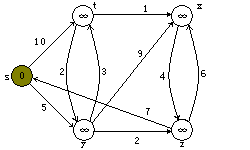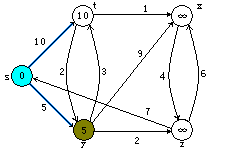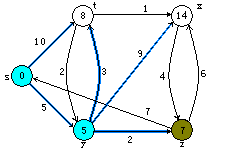a b c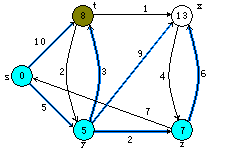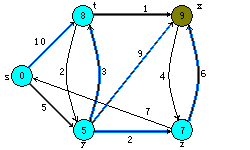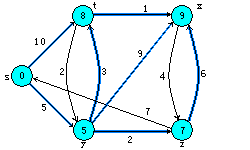d e f

Dijkstra算法的执行过程。源点s为最坐端顶点。最短路径估计被标记在顶点内，阴影覆盖的边指出了前趋的值。 蓝色顶点在集合S中，而白色顶点在最小优先级队列Q=V-S中。 a)第4～8行while循环第一次迭代前的情形。阴影覆盖的顶点具有最小的d值，而且在第5行被选为顶点u。 b)至f)while循环在每一次连续迭代后的情形。每个图中阴影覆盖的顶点被选作下一次迭代第5行的顶点u。 f)图中的值为最终结果。

# 算法实现

``````struct node {
int ord;
int cost;
} v;

for (i = len; i; i--) {
make_heap(v + 1, v + 1 + i);
for (j = 2; j <= i; j++) {
if (v[j].cost > v.cost + mp[v.ord][v[j].ord])
v[j].cost = v.cost + mp[v.ord][v[j].ord];
}
swap(v, v[i]);
}
sort(v + 1, v + 1 + len, cmp);
``````

# 参考源码

redraiment使用Emacs Lisp批量迁移《HDU 2060-2069》